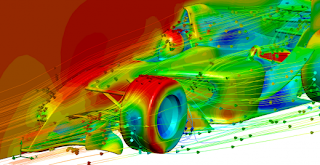## Wednesday, June 09, 2010

### Initial thoughts on turbulenceWhilst a general theory of turbulence continues to elude mathematical physics, the phenomenon can actually be characterised in rather simple terms:

Turbulence is an intermediate state of a fluid between laminar flow and thermodynamic equilibrium.

Thermodynamic equilibrium is the state of maximum entropy, the state in which no information is embedded in the fluid. In such a state, all the energy that may once have been carried by the streamlines of the fluid, has been dissipated into thermal energy, the random heat energy of the molecules in the fluid. The direction of motion of the molecules in thermodynamic equilibrium is isotropically distributed, and the speed of motion of the molecules possesses the Maxwell-Boltzmann distribution.

In contrast, laminar flow is a low-entropy state of a fluid, in which the fluid carries both directional information, and speed of motion information, defined by the streamlines and trajectories of the fluid flow velocity field.

Turbulent flow is intermediate between laminar flow and thermodynamic equilibrium because it constitutes a flow regime in which the directional information of laminar flow has been degraded, but the speed of motion information has been at least partially retained. In this respect, turbulent flow is defined by its possession of the following two characteristics:

(i) Chaotic motion.
(ii) Vorticity.

In chaotic motion, the distance between a pair of particle trajectories, initially close together, can diverge as a function of time. This property destroys the 'parallel' nature of the particle trajectories in laminar flow.

'Vorticity' is the fancy word for rotation in a fluid, and turbulent flow is typically characterised by a cascade of vortices of different sizes. Each vortex transfers its angular momentum to vortices of a smaller size, which then, in turn, transfer their angular momentum to yet smaller vortices. (In the case of turbulent aerodynamics, one can think of the cascade as a type of pneumatic clockwork mechanism). The smallest vortices are sufficiently small that the viscosity of the fluid is able to transform their rotational energy into heat energy.

Chaotic motion and vorticity both clearly degrade the directional information carried by laminar fluid flow. However, the distribution of particle speeds carried by the laminar flow can be partially preserved in turbulent motion. Hence, it is in this sense that turbulent motion is an intermediate state between laminar flow and thermodynamic equilibrium.